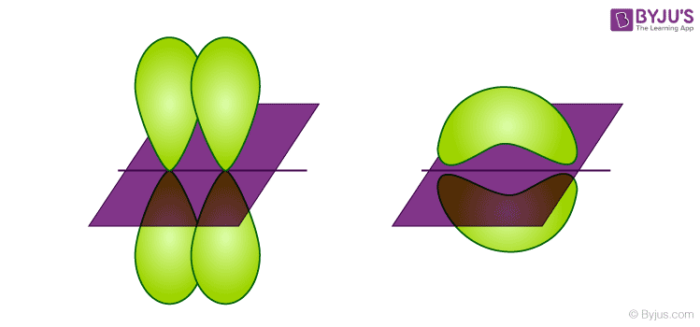# Conditions for LCAO (Linear Combination of Atomic orbitals)### What is meant by LCAO (Linear Combination of Atomic orbitals)?

The Linear combination of atomic orbitals which is also known as LCAO is an approximate method for representing molecular orbitals. It’s more of a superimposition method where constructive interference of two atomic wave functions produces a bonding molecular orbital whereas destructive interference produces non-bonding molecular orbital.

### Conditions to be satisfied:

The conditions that are required for a linear combination of atomic orbitals are as follows:

• Same Energy of combining orbitals – The combining atomic orbitals must have the same or nearly the same energy. This means that the 2p orbital of an atom can combine with another 2p orbital of another atom but 1s and 2p cannot combine as they have appreciable energy difference.
• Same symmetry about the molecular axis – The combining atoms should have the same symmetry around the molecular axis for proper combination, otherwise, the electron density will be sparse.
For e.g. all the sub-orbitals of 2p have the same energy but still, the 2pz orbital of an atom can only combine with a 2pz orbital of another atom but cannot combine with 2px and 2py orbital as they have a different axis of symmetry.
In general, the z-axis is considered as the molecular axis of symmetry.
• Proper Overlap between the atomic orbitals – The two atomic orbitals will combine to form molecular orbital if the overlap is proper. Greater the extent of overlap of orbitals, greater will be the nuclear density between the nuclei of the two atoms.

The condition can be understood by two simple requirements. For the formation of the proper molecular orbital, proper energy and orientation are required. For proper energy, the two atomic orbitals should have the same energy of orbitals and for the proper orientation, the atomic orbitals should have proper overlap and the same molecular axis of symmetry.

For more information about conditions for LCAO (Linear Combination of Atomic orbitals), visit BYJU’S.

Test your knowledge on Linear Combination Of Atomic Orbitals Conditions